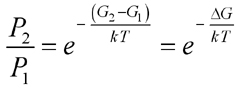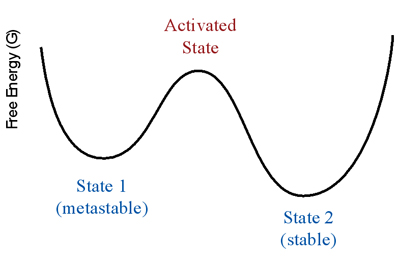# Entropy Activity

-Introduction

-The reaction coordinate

-The energy landscape

-Population distributions

-Motion at constant temperature, and exchange of energy with the heat bath

-Thermally activated processes

-Energy and temperature determine the populations

-Entropy and free energy

-Mathematical derivation of free energy

 < Previous | Next >

## Entropy and free energy

This simulation introduces entropy into the systems. The simulation extends the above two-dimensional boxes to three dimensions.

Virtual Activity

- Entropy and free energy [Opens in a separate window.]

You should have found that at high temperature, more boxes are standing up and most of the balls are on the upper platforms. Why does most of the population move to the higher-energy state? The concept we use to explain this is entropy. The summary explanation is: "Although energy prefers that the boxes lie down, entropy prefers that they stand up. At low temperature, energy wins and most boxes are lying down. At high temperature, entropy wins and more boxes are standing up." We will now examine this explanation in more detail.

Entropy refers to the number of ways a system can be in a certain state. For the above rectangular box there are 4 ways to have high energy and only 2 ways to have low energy, so the entropy is larger in the higher energy state. We can summarize this with the following table: (We do not need to make a distinction between energy and enthalpy here, and so will use E and H interchangeably.)

 Energy (E or H) Entropy (S) Standing-up boxes/higher platforms favored Laying-down boxes/lower platforms favored

Or, equivalently, we can say the following reactions:

box laying down   =>   box standing up
ball on lower 2 platforms   =>   ball on upper 4 platforms

are entropy-driven but not enthalpy-driven.

This interplay of energy and entropy is captured mathematically by the free energy:

G = H - TS

Systems always favor the state with the lowest free-energy. We can understand the above expression for G as follows. Since the entropy is multiplied by temperature, T, entropy becomes more important at high temperature. The energy and entropy terms have opposite sign (E vs. -TS) because systems prefer low energy and high entropy. At low temperature, energy (H) dominates the free energy: most of the boxes are lying down and most of the balls are on the lower platforms. At sufficiently high temperature, entropy (-TS) begins to dominate: most of the boxes are standing up and most of the balls are on the upper platform.

We will show on the next page that we can include the entropy effects by replacing energy with free energy in our population expression:We include this in our reaction path diagram by labelling the y-axis with free energy, G, instead of energy, E.< Previous | Next >